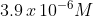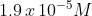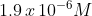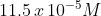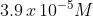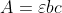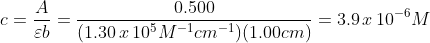# AP Chemistry : Spectroscopy and Electromagnetic Spectrum

## Example Questions

### Example Question #114 : Solutions And States Of Matter

What is the energy of a photon that has a wavelength of 540 nm?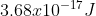None of the above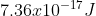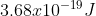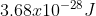Explanation: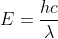;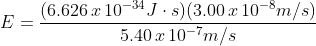;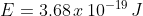### Example Question #115 : Solutions And States Of Matter

What transition would emit the longest wavelength of light?

n = 3 to n = 1

Not enough information given

n = 4 to n = 1

n = 4 to n = 3

n = 2 to n = 1

n = 4 to n = 3

Explanation:

Wavelength is inversely proportional to energy.  Since the transition from n=4 to n=3 is the lowest energy transition, it would have the longest wavelength.

### Example Question #116 : Solutions And States Of Matter

What photon wavelength can promote a transition from the n = 1 (ground state) to the n = 3 (excited state)?

306 nm

102 nm

204 nm

408 nm

1 m

102 nm

Explanation: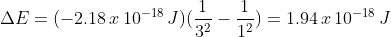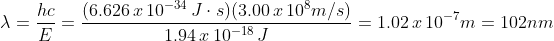### Example Question #111 : Solutions And States Of Matter

A 3.0 eV light is shined on Gold ( Փ = 5.1 eV), silver (Փ =4.26 eV), Cesium (Փ = 2.1 eV), and Platinum (Փ = 6.35).  If Փ is the work function of the metal, what metal would have electrons ejected?

Pt

Ag

Cs

Au, Ag, and Pt

Au

Cs

Explanation:

The work function is the energy required to remove an electron from a metal.  Only Cs metal has a work function less than the energy of the light.

### Example Question #118 : Solutions And States Of Matter

A student has a Blue #1 dye with a molar absorptivity of 1.30 x 105 M-1 cm-1 at 631 nm.  If the student runs this sample through a spectrophotometer and finds it has an absorption of 0.500 what is the dye’s molarity?  Assume a path length of 1.0 cm.